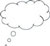# 4 Modelling a context mathematically

Word problems are often essentially mathematical problems dressed up in everyday language. They can help students understand that mathematics does indeed relate to the real world, and that they themselves are acting like mathematicians when they decode word problems. This is why it is important that students grasp the concept that mathematics in real-world problems models complex situations and they need to be confident and knowledgeable enough to extract the essential elements from them.

Focusing on the process of making sense of a complex situation and modelling it mathematically can also help students to focus on the ‘making sense’ aspect of word problems.

Identifying the mathematical ideas that will be needed to solve word problems, or modelling the context mathematically, can be difficult for students. The word problems they encounter in textbooks usually only need the mathematics that has just been studied – so thinking more broadly about what other mathematics may be needed to model the situation is seldom required, except in examinations. The next activity focuses on students thinking about what mathematics is needed and how to express the problem in a way that mathematics can be used to solve the problem. Read the activity and case study, and then plan and carry out the activity with your class.

## Activity 3: Identifying the mathematical model for a word problem

Here are three word problems:

1. Yamini and Fatima, two Class IX students, together contributed Rs. 100 towards the Prime Minister’s Relief Fund to help the earthquake victims. Write a linear equation that matches this text.
2. Mary wants to decorate her Christmas tree. She wants to place the tree on a wooden box covered with coloured paper with picture of Santa Claus on it. She must know the exact quantity of paper to buy for this purpose. If the box is 80 cm long, 40 cm wide and 20 cm high, how many 40 cm square sheets of paper would she require?
3. The Shanti Sweets Stall was placing an order for making cardboard boxes for packing its sweets. Two sizes of boxes were required: a larger box with dimensions of 25 cm × 20 cm × 5 cm, and a smaller box with dimensions of 15 cm × 12 cm × 5 cm. For all the overlaps, 5 per cent of the total surface area is required extra. If the cost of the cardboard is Rs. 4 for 1000 cm2, find the cost of cardboard required for supplying 250 boxes of each kind.

For each word problem given:

• draw an illustration for the problem
• identify the unknowns in the problems
• identify what you know
• find the relationship between the unknowns and knowns
• represent the relationship mathematically.

## Case Study 3: Mrs Rawool reflects on Activity 3

I showed the class the three problems from Activity 3 and asked them not to solve them, but to work through the five steps in modelling the mathematics in the situation. Of course, several of the students set about solving the problems: when they put up their hands to give the answer, I asked them if they had a question and to stand up so that the whole class could hear it and help answer it. That confused them a bit until I reminded them about what they were supposed to be doing!Pause for thought Think about the pedagogical approach of ‘no hands up’: did your students put their hands up with an answer to the problem when you had asked them to think about the process? Do you think a ‘no hands up’ rule except to ask a question, would help encourage your students to work more collaboratively and therefore do more thinking and learning?